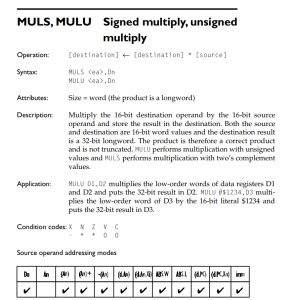# Fixed point number formats: might use 16.16So for my fixed point number format for my 3D project, I’ve come up with a few possibilities :

• I think a 16.16 number format might work for me. This means I’ll represent the whole portion via one 68k word, and the fractional portion with one 68K word.
• It would look like this: <Sign-bit><15-bit integer>.<16-bit integer>
• with using 0 for Sign-bit if the number is positive, and 1 if the number is negative
• This allows me to use standard multiplication instructions, like muls.w on the portions that require it. The instruction will do the sign handling.
• My first approach of multiplying these numbers together would be to use the distributive property of multiplication such that you’d end up with:
• (a+b) The whole part + the fractional part of the first number
• (c+d) The whole part + the fractional part of the second number
• So, (a+b)(c+d), would take (4) multiplications, (3) muls.w and a (1) mulu.w.
• This sounds super expensive and just inelegant. Gotta be a better way.
• I’m thinking I can use regular addition and subtraction, with adding/subtracting 1 to the whole part if the fractional part overflows/goes negative. I’ll have to figure out how the CCR flags react in those cases.

I’m definitely going down the path of working this out. What I really need is an easy way to set multiple memory locations in bulk based on a file. The monitor software I’m using only allows for accessing 64K of RAM, I’m either going to have to extend it, or find something else that will take an SREC or similar format, and allow me to upload it.